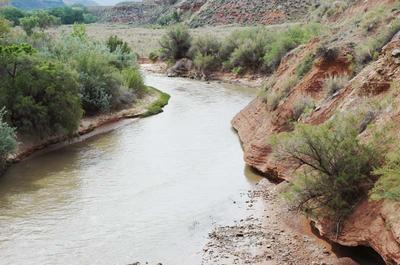online_all_series_uh_cascade:   All series of dimensionless CRL unit hydrographFormulas C1 = C/(2+C)           C2 = (2-C)/(2+C) Qj+1n+1 = 2C1(Qaverage)j + C2Qj+1n
 Reference

 RATIONALE This program calculates a set of dimensionless unit hydrographs (DUH) by the cascade of linear reservoirs (CLR), for the following six (6) values of Courant number: 2, 1.5, 1, 0.5, 0.2, and 0.1; and the number of reservoirs N varying from 1 to 10. The Courant number is C = tr/ K. The value tr is the unit hydrograph duration, in hours. The value K is the reservoir storage constant, in hours. The program can be used to compare amounts of runoff diffusion within the given (practical) range of C and N values. The dimensionless unit hydrograph has dimensionless time t* as abscissa and dimensionless discharge Q* as ordinate. The dimensionless time is t* = t / tr, in which tr is the duration of the unit hydrograph, in hours. The dimensionless discharge is Q* = Q / Qm, in which Qm = 2.777778 (A / tr), is the maximum attainable discharge (runoff concentration only), in m3/s. In this formula, basin area A is in km2. The program provides six DUH tables of Q* vs t*, for values of Courant number: 2, 1.5, 1, 0.5, 0.2, and 0.1. A summary table of CLR DUH peak discharge and time of occurrence precedes the six DUH tables. Note that the largest value of DUH peak discharge is Q* = 1 at time t* = 1, associated with C = 2 and N = 1. This is the least diffused DUH. Also, note that the smallest value of DUH peak discharge is Q* = 0.013 at time t* = 91, associated with C = 0.1 and N = 10. This is the most diffused DUH.

Thank you for running online_all_series_uh_cascade.